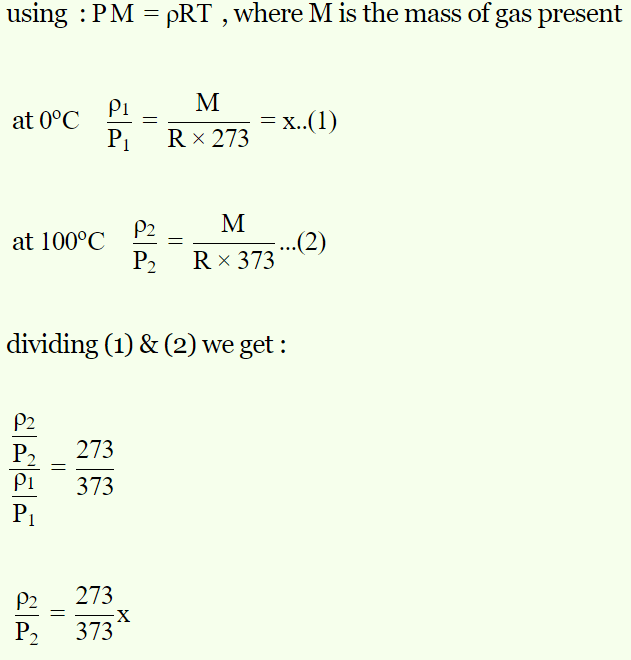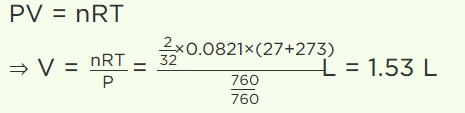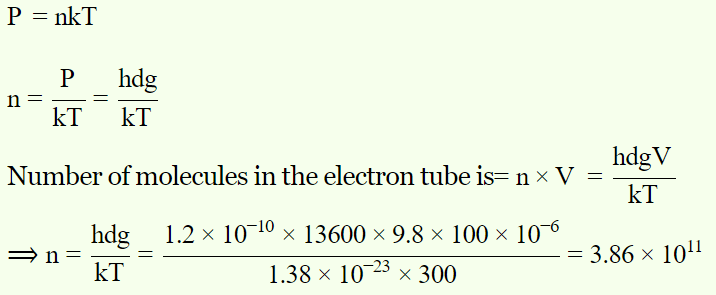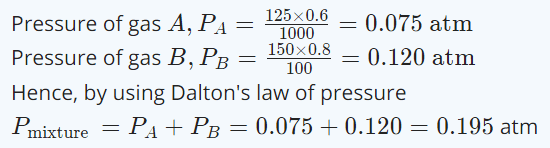## Kinetic Theory Questions and Answers Part-8

1. The value of critical temperature in terms of Vander Waal’s constant a and b is
a) $T_{C}=\frac{8a}{27Rb}$
b) $T_{C}=\frac{a}{2Rb}$
c) $T_{C}=\frac{8}{27Rb}$
d) $T_{C}=\frac{27a}{8Rb}$

Explanation: The value of critical temperature in terms of Vander Waal’s constant a and b is $T_{C}=\frac{8a}{27Rb}$

2. In Vander Waal’s equation a and b represent $\left(P+\frac{a}{V^{2}}\right)\left(V-b\right)=RT$
a) Both a and b represent correction in volume
b) Both a and b represent adhesive force between molecules
c) a represents adhesive force between molecules and b correction in volume
d) a represents correction in volume and b represents adhesive force between molecules

Explanation: a represents adhesive force between molecules and b correction in volume

3. The gas in vessel is subjected to a pressure of 20 atmosphere at a temperature 27°C. The pressure of the gas in a vessel after one half of the gas is released from the vessel and the temperature of the remainder is raised by 50°C is
a) 8.5 atm
b) 10.8 atm
c) 11.7 atm
d) 17 atm

Explanation:4. At 0°C the density of a fixed mass of a gas divided by pressure is x. At 100°C, the ratio will be
a) x
b) $\frac{273}{373}x$
c) $\frac{373}{273}x$
d) $\frac{100}{273}x$

Explanation:5. 2 gm of $O_{2}$ gas is taken at 27°C and pressure 76 cm. Of Hg. Then find out volume of gas (in litre)
a) 1.53
b) 2.44
c) 3.08
d) 44.2

Explanation:6. An electron tube was sealed off during manufacture at a pressure of $1.2 \times10^{ -7}mm$    of mercury at 27°C. Its volume is 100 cm3. The number of molecules that remain in the tube is
a) $2 \times10^{ 16}$
b) $3\times10^{ 15}$
c) $3.86 \times10^{11}$
d) $5 \times10^{11}$

Explanation:7. If the pressure of an ideal gas contained in a closed vessel is increased by 0.5%, the increase in temperature is 2K. The initial temperature of the gas is
a) 27°C
b) 127°C
c) 300°C
d) 400°C

Explanation: If the pressure of an ideal gas contained in a closed vessel is increased by 0.5%, the increase in temperature is 2K. The initial temperature of the gas is 127°C

8. Air is filled in a bottle at atmospheric pressure and it is corked at 35°C. If the cork can come out at 3 atmospheric pressure than upto what temperature should the bottle be heated in order to remove the cork
a) 325.5°C
b) 851°C
c) 651°C
d) None of these

Explanation:9. A gas is collected over the water at 25°C. The total pressure of moist gas was 735 mm of mercury. If the aqueous vapour pressure at 25°C is 23.8 mm. Then the pressure of dry gas is
a) 760 mm
b) 758.8 mm
c) 710.8 mm
d) 711.2 mm